Reconstruction of the 2020 Ackling Dyke formation 1.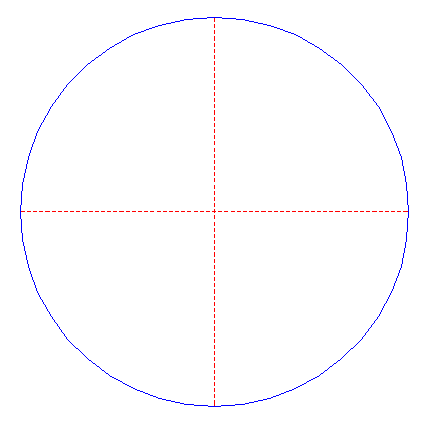Draw a circle. Draw the horizontal and vertical centerlines. 2.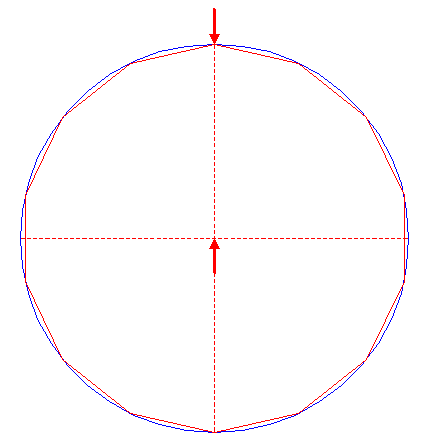Construct the inscribed regular 14-sided polygon of circle 1, pointing up. 3.Draw the fourteen rays of polygon 2, from the center to the angular points. Number these rays clockwise 1 - 14, starting at the top. 4.Draw the connecting line between the righthand intersection of circle 1 and the horizontal centerline, and the intersection of circle 1 and ray 3 nr. 1. 5.Construct a circle concentric to circle 1, tangent to line 4. 6.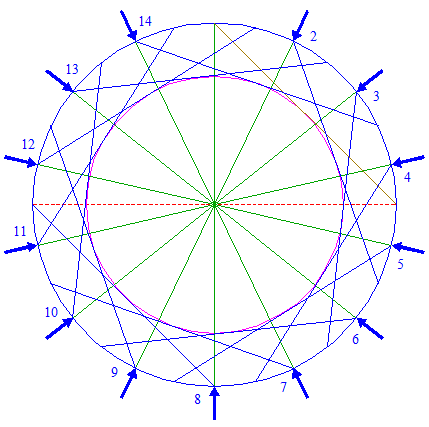Repeat step 4 thirteen times, with respect to the other rays 3 and corresponding positions, as shown. 7.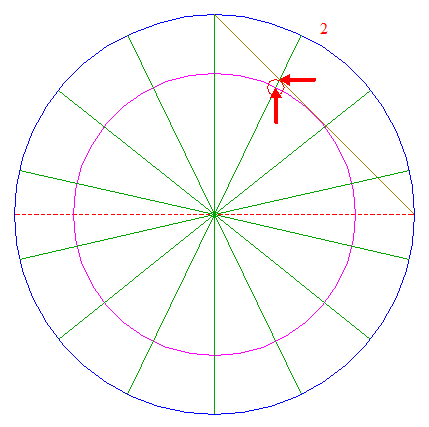Construct a circle centered at the intersection of circle 5 and ray 3 nr. 2, passing through the intersection of line 4 and ray 3 nr. 2. 8.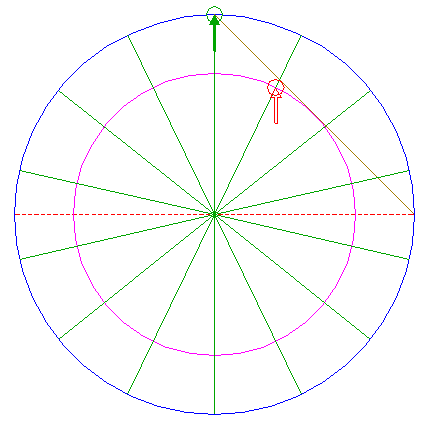Copy circle 7 to the upper intersection of circle 1 and the vertical centerline. 9.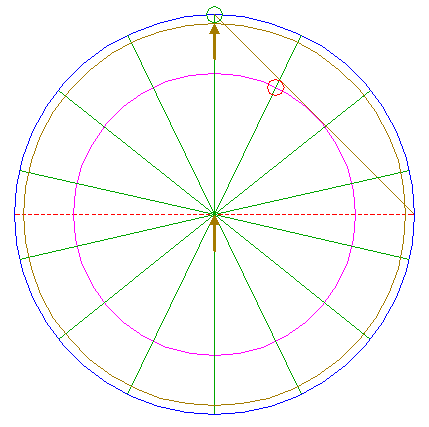Construct a circle concentric to circle 1, tangent to circle 8 at the lower side. 10.Draw the connecting line between the righthand intersection of circle 1 and the horizontal centerline, and the intersection of circle 9 and ray 3 nr. 14. 11.Construct a circle concentric to circle 1, tangent to line 10. 12.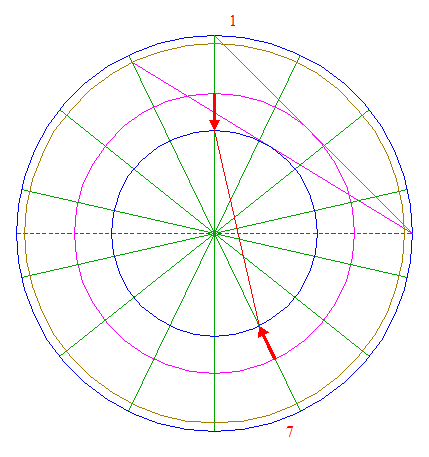Draw the connecting line between the intersections of circle 11 with rays 3 nrs. 1 and 7. 13.Repeat step 12 six times, with respect to the pairs of rays 3 nrs. 3 and 9, 5 and 11, 7 and 13, 9 and 1, 11 and 3, and 13 and 5. 14.Circles 1, 5, and 9, and lines 3, 4, 6, 12, and 13, are used for the final reconstruction. 15.Remove all parts not visible within the formation itself. 16.Colour all areas corresponding to standing... 17....or to flattened crop, and finish the reconstruction of the 2020 Ackling Dyke formation. 18.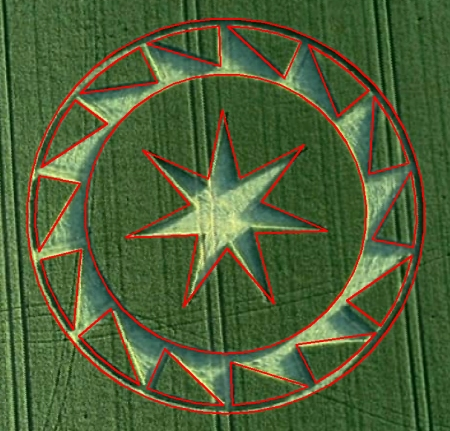The final result, matched with the aerial image.